# Knowledge Base

## Solution 11975: Percent (%) Operator on the TI-83 Family, TI-84 Plus Family, and TI-Nspire™ in TI-84 Plus Mode.

### Do the TI-83 family, TI-84 Plus family, or TI-Nspire in TI-84 Plus mode make use of a percent operator (%)?

The TI-83 family, TI-84 Plus family, or TI-Nspire in TI-84 Plus mode do not include a percent operator (%). Therefore, in order to convert a number to a percentage, simply divide that number by 100. Likewise, to convert a percentage to a number, multiply that number by 100. The following examples demonstrate exactly how to perform some percentage-related calculations:

Example - Percentage: Calculate 5% of 250.

• Press  [÷]    [ENTER] to find the percentage in decimal format.
• Press    [×] [.]   [ENTER].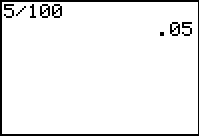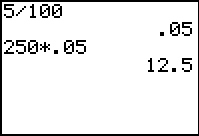Example - Ratio: Calculate the ratio of 250 to 5.

• Press    [÷] [.]   [ENTER].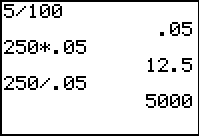• Press    [×]  [.]   [ENTER].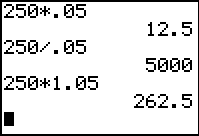Example - Discount: Calculate a 5% discount on 250.

• Press   [÷]    to find the percentage after discount.
• Press    [×] [.]   [ENTER].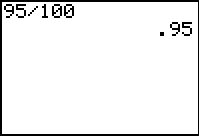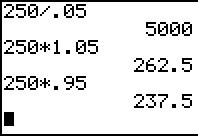Please see the TI-83 family and TI-84 Plus family guidebooks for additional information.

Users with the TI-Nspire in TI-84 Plus mode may use the TI-84 Plus guidebook.